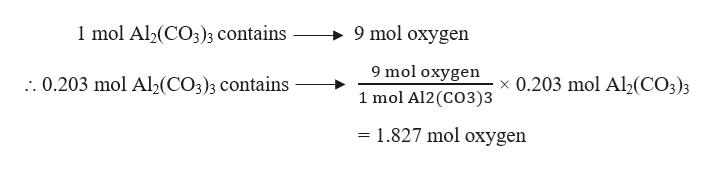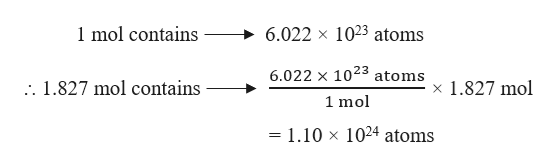# How many atoms of oxygen are contained in 47.6 g of Al2(CO3)3? The molar mass of Al2(CO3)3 is 233.99 g/mol.

Question
245 views

How many atoms of oxygen are contained in 47.6 g of Al2(CO3)3? The molar mass of Al2(CO3)is 233.99 g/mol.

check_circle

Step 1

Given,

Mass of Al2(CO3)3 = 47.6 g

Molar mass of Al2(CO3)= 233.99 g/mol

Number of moles of Al2(CO3)can be calculated as:

Step 2

1 mol of Al2(CO3)contains 3×3 = 9 mol of oxygen. Therefore, moles of oxygen in 0.203 mol of Al2(CO3)can be calculated as :help_outlineImage Transcriptionclose9 mol oxygen 1 mol Al2(CO3)3 contains 9 mol oxygen x 0.203 mol Al2(CO3)3 .. 0.203 mol Al2(CO3)3 contains 1 mol Al2(CO3)3 = 1.827 mol oxygen fullscreen
Step 3

The number of atoms of in 1.827 mol o...help_outlineImage Transcriptionclose6.022 x 1023 atoms 1 mol contains 6.022 x 1023 atoms x 1.827 mol . 1.827 mol contains 1 mol 1024 atoms = 1.10 x fullscreen

### Want to see the full answer?

See Solution

#### Want to see this answer and more?

Solutions are written by subject experts who are available 24/7. Questions are typically answered within 1 hour.*

See Solution
*Response times may vary by subject and question.
Tagged in

### Chemistry